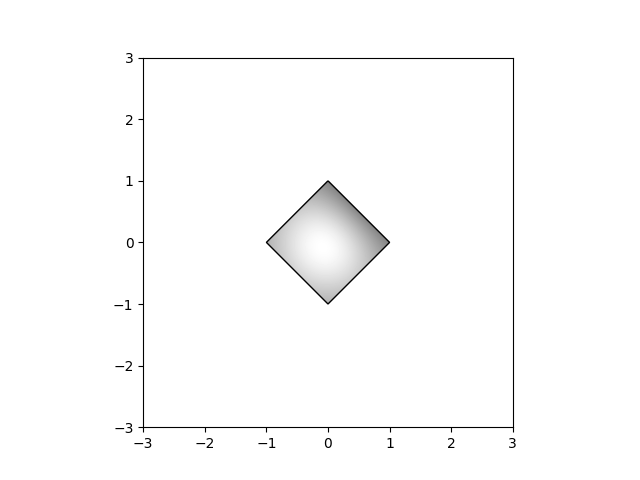Image Demo¶

Many ways to plot images in Matplotlib.

The most common way to plot images in Matplotlib is with imshow. The following examples demonstrate much of the functionality of imshow and the many images you can create.

from __future__ import print_function

import numpy as np
import matplotlib.cm as cm
import matplotlib.pyplot as plt
import matplotlib.cbook as cbook
from matplotlib.path import Path
from matplotlib.patches import PathPatch

First we’ll generate a simple bivariate normal distribution.

delta = 0.025
x = y = np.arange(-3.0, 3.0, delta)
X, Y = np.meshgrid(x, y)
Z1 = np.exp(-X**2 - Y**2)
Z2 = np.exp(-(X - 1)**2 - (Y - 1)**2)
Z = (Z1 - Z2) * 2

im = plt.imshow(Z, interpolation='bilinear', cmap=cm.RdYlGn,
origin='lower', extent=[-3, 3, -3, 3],
vmax=abs(Z).max(), vmin=-abs(Z).max())

plt.show()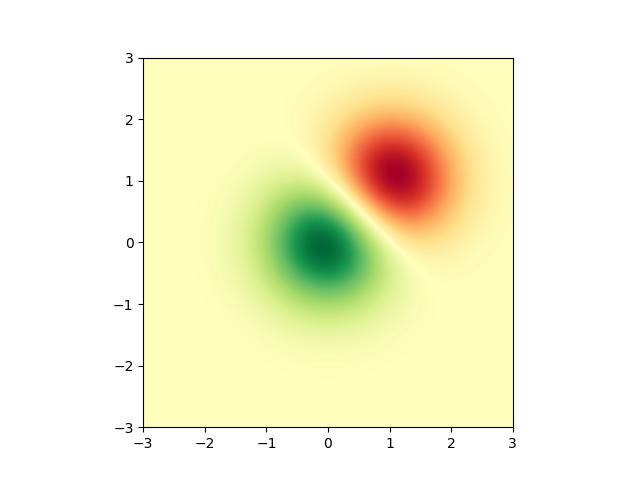It is also possible to show images of pictures.

# A sample image
with cbook.get_sample_data('ada.png') as image_file:

fig, ax = plt.subplots()
ax.imshow(image)
ax.axis('off')  # clear x- and y-axes

# And another image

w, h = 512, 512

with cbook.get_sample_data('ct.raw.gz', asfileobj=True) as datafile:
A = np.fromstring(s, np.uint16).astype(float).reshape((w, h))
A /= A.max()

fig, ax = plt.subplots()
extent = (0, 25, 0, 25)
im = ax.imshow(A, cmap=plt.cm.hot, origin='upper', extent=extent)

markers = [(15.9, 14.5), (16.8, 15)]
x, y = zip(*markers)
ax.plot(x, y, 'o')

ax.set_title('CT density')

plt.show()
••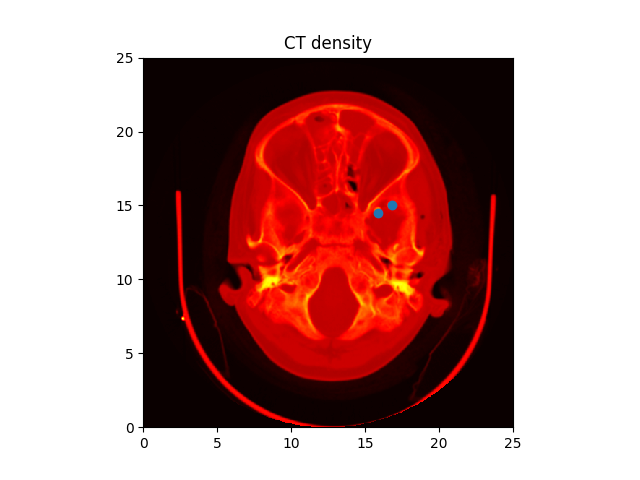Interpolating images¶

It is also possible to interpolate images before displaying them. Be careful, as this may manipulate the way your data looks, but it can be helpful for achieving the look you want. Below we’ll display the same (small) array, interpolated with three different interpolation methods.

The center of the pixel at A[i,j] is plotted at i+0.5, i+0.5. If you are using interpolation=’nearest’, the region bounded by (i,j) and (i+1,j+1) will have the same color. If you are using interpolation, the pixel center will have the same color as it does with nearest, but other pixels will be interpolated between the neighboring pixels.

Earlier versions of matplotlib (<0.63) tried to hide the edge effects from you by setting the view limits so that they would not be visible. A recent bugfix in antigrain, and a new implementation in the matplotlib._image module which takes advantage of this fix, no longer makes this necessary. To prevent edge effects, when doing interpolation, the matplotlib._image module now pads the input array with identical pixels around the edge. e.g., if you have a 5x5 array with colors a-y as below:

a b c d e
f g h i j
k l m n o
p q r s t
u v w x y

the _image module creates the padded array,:

a a b c d e e
a a b c d e e
f f g h i j j
k k l m n o o
p p q r s t t
o u v w x y y
o u v w x y y

does the interpolation/resizing, and then extracts the central region. This allows you to plot the full range of your array w/o edge effects, and for example to layer multiple images of different sizes over one another with different interpolation methods - see examples/layer_images.py. It also implies a performance hit, as this new temporary, padded array must be created. Sophisticated interpolation also implies a performance hit, so if you need maximal performance or have very large images, interpolation=’nearest’ is suggested.

A = np.random.rand(5, 5)

fig, axs = plt.subplots(1, 3, figsize=(10, 3))
for ax, interp in zip(axs, ['nearest', 'bilinear', 'bicubic']):
ax.imshow(A, interpolation=interp)
ax.set_title(interp.capitalize())
ax.grid(True)

plt.show()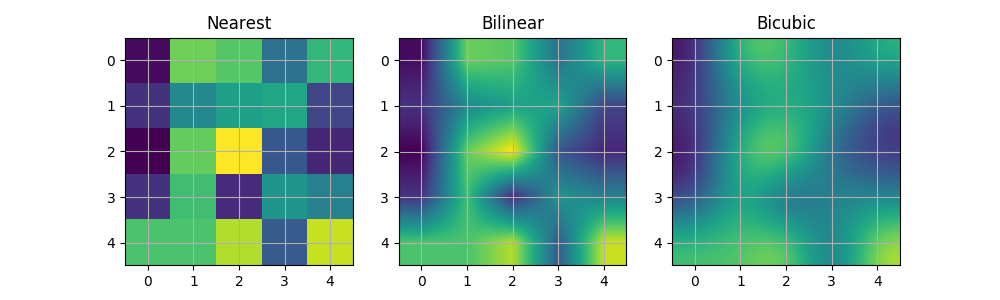You can specify whether images should be plotted with the array origin x[0,0] in the upper left or lower right by using the origin parameter. You can also control the default setting image.origin in your matplotlibrc file

x = np.arange(120).reshape((10, 12))

interp = 'bilinear'
fig, axs = plt.subplots(nrows=2, sharex=True, figsize=(3, 5))
axs.set_title('blue should be up')
axs.imshow(x, origin='upper', interpolation=interp)

axs.set_title('blue should be down')
axs.imshow(x, origin='lower', interpolation=interp)
plt.show()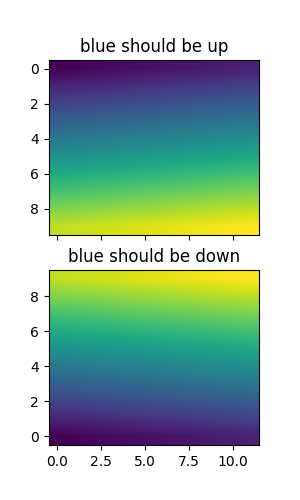Finally, we’ll show an image using a clip path.

delta = 0.025
x = y = np.arange(-3.0, 3.0, delta)
X, Y = np.meshgrid(x, y)
Z1 = np.exp(-X**2 - Y**2)
Z2 = np.exp(-(X - 1)**2 - (Y - 1)**2)
Z = (Z1 - Z2) * 2

path = Path([[0, 1], [1, 0], [0, -1], [-1, 0], [0, 1]])
patch = PathPatch(path, facecolor='none')

fig, ax = plt.subplots()